# Cyclist

Cyclist goes uphill 10 km for 50 minutes and downhill minutes for 29 minutes, both applied to the pedals same force. How long he pass 10 km by plane?

Result

t =  36.7 min

#### Solution:

$\dfrac{1}{ 50}+\dfrac{1}{ 29} = \dfrac{2}{ t} \ \\ \ \\ t = \dfrac { 2\cdot 50\cdot 29}{ 50+29 } = 36.7 \ \text{min}$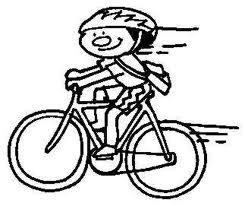Our examples were largely sent or created by pupils and students themselves. Therefore, we would be pleased if you could send us any errors you found, spelling mistakes, or rephasing the example. Thank you!

Leave us a comment of this math problem and its solution (i.e. if it is still somewhat unclear...):Be the first to comment!Tips to related online calculators
Looking for calculator of harmonic mean?
Looking for a statistical calculator?
Need help calculate sum, simplify or multiply fractions? Try our fraction calculator.
Check out our ratio calculator.

## Next similar math problems:

1. Uphill and downhillThe cyclist moves uphill at a constant speed of v1 = 10 km/h. When he reaches the top of the hill, he turns and passes the same track downhill at a speed of v2 = 40 km/h. What is the average speed of a cyclist?
2. Two cyclists 2At the same time, two cyclists left the towns A and B at constant speeds. The first one going from town A to town B, and the second one from town B to town A. At one point of the trip they met. After they met, the first cyclist arrived at town B in 36min,
3. Two citiesThe car goes from city A to city B at an average speed of 70 km/h, back at an average speed of 50 km/h. If it goes to B and back at an average speed of 60 km/h, the whole ride would take 8 minutes less. What is the distance between cities A and B?
4. Ten cashiers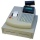Ten cashiers are open at Tesco. Customers wait an average of 15 minutes. How many other cashiers have to open to reduce the waiting time by 4 minutes?
5. Flowerbed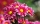Ondra digs the bed in 20 minutes. Cuba in 30 minutes. For how long do they dig together?
6. Two pumps togetherThe first pump will fill the tank itself in 3 hours and the second one in 6 hours. How many hours will the tank be full if both pumps are worked at the same time?
7. Aircraft angines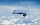The two engines of the aircraft are enough to supply the fuel for five hours of operation. However, one of the engines has a malfunction and thus consumes one-third more fuel. How long can the plane be in the air before it runs out of fuel? After an hour
8. Panel house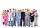The construction company has two groups of workers. Group A has more members than B. Panel house was insulated by group A in 10 days. The same block of flats was insulated together in 6 days. How many days would group B block insulate?
9. Mixture of nutsThe mixture of nuts should be prepared from almonds, peanuts and cashew nuts ratio 1: 2: 3 (respectively). The price of almonds is 150 CZK/kg, the price of peanuts is 140 CZK/kg and the price of cashew nuts is 180 CZK/kg. The price of the mixture is deter
10. Between two bus stops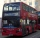Wanda lives between two bus stops at three-eighths of their distance. He started the house today and found that whether he was running to one or the other stop, he would have arrived at the bus stop. The average bus speed is 60 km/h. What is the average
11. Average speedThe truck drove 1/2 of the way on the highway at 80km/h. The other half of the way 20km/h. Calculate the average speed
12. Three altitudes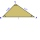A triangle with altitudes 4; 5 and 6 cm is given. Calculate the lengths of all medians and all sides in a triangle.Lucy, Tereza, and Petra decided to compile the compulsory reading records together. It would take 30 days for Lucia alone, 36 for Tereza and 45 for Petra. How long will it take to make records if they will work together?Four pavers would pave the square in 18 days. How many pavers do you need to add to done work in 12 days?In a 400 meter athletic competition, a participant covers the distance as given below. find the average speed? first 80 meters 10 m/s next 240 meters 7.5 m/s last 80 meters 10 m/sCarpenters 1 and 2 spend 10 days and 5 days respectively to make one table. If 50 tables were made by the first carpenter and 30 tables were made by the second carpenter, What is the average time spent on the products?Two cities along the river are 100 km apart. The powerboat downstream runs for 4 hours, upstream for 10 hours. Determines the river's current speed.